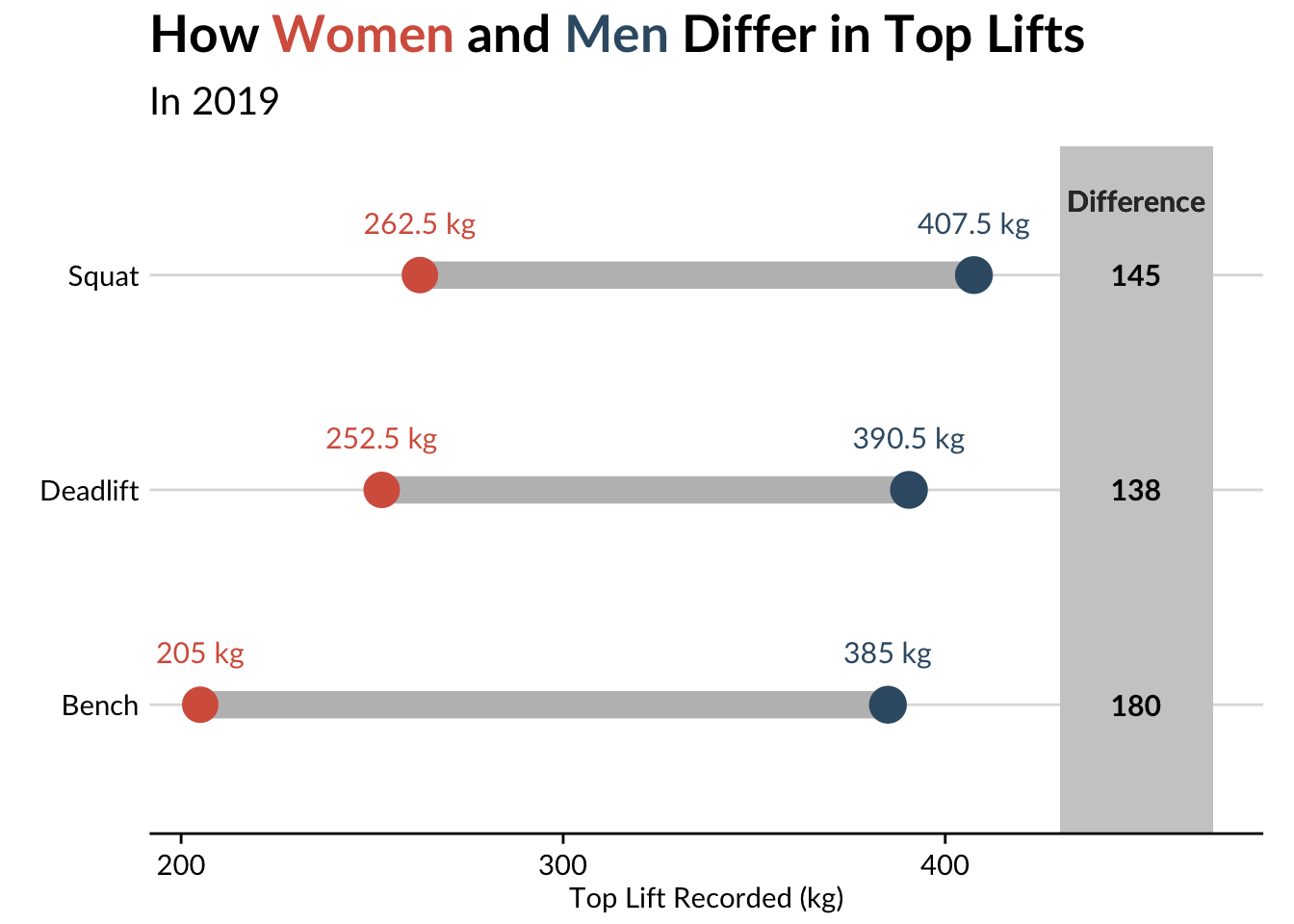``````library(ggplot2)
library(tidyverse)
library(cr)

set_cr_theme(font = "lato")``````

First, read in the data from https://openpowerlifting.org/data:

``````# df <- readr::read_csv("openpowerlifting-2019-09-20.csv")
#
# df_clean <- df %>%
#   janitor::clean_names()
#
# ipf_lifts <- df_clean %>%
#   select(name:weight_class_kg, starts_with("best"), place, date, federation, meet_name)  %>%
#   filter(!is.na(date)) %>%
#   filter(federation == "IPF")

Clean ipf_lifts, and reshape the three lifts into one column:

``````ipf_lifts <- ipf_lifts %>%
mutate(year = lubridate::year(date))

ipf_lifts_reshape <- ipf_lifts %>%
tidyr::pivot_longer(cols = c("best3squat_kg", "best3bench_kg", "best3deadlift_kg"), names_to = "lift") %>%
select(name, sex, year, lift, value)``````

For my visualization, I’m only concerned with the heaviest lifts from each year:

``````ipf_lifts_maxes <- ipf_lifts_reshape %>%
group_by(year, sex, lift) %>%
top_n(1, value) %>%
ungroup %>%
distinct(year, lift, value, .keep_all = TRUE)``````

In order to construct a dumbbell plot, we need both male and female observations in the same row.

``````max_pivot <- ipf_lifts_maxes %>%

Let’s try to construct a dataframe for each sex:

``````male_lifts <- max_pivot %>%
select(-name) %>%
filter(!is.na(M)) %>%
group_by(year, lift) %>%
summarise(male = mean(M))

female_lifts <- max_pivot %>%
select(-name) %>%
filter(!is.na(`F`)) %>%
group_by(year, lift) %>%
summarise(female = mean(`F`))``````

And join them:

``````max_lifts <- merge(male_lifts, female_lifts)

max_lifts_final <- max_lifts %>%
group_by(year, lift) %>%
mutate(diff = male - female)``````

## Visualize

Finally, we can construct the visualization.

First, a static viz (thanks to hrbrmaster’s `ggalt` package):

``````library(ggtext)
max_lifts_final %>%
filter(year == 2019) %>%
ggplot() +
ggalt::geom_dumbbell(aes(y = lift,
x = female, xend = male),
colour = "grey", size = 5,
colour_x = "#D6604C", colour_xend = "#395B74") +
labs(y = element_blank(),
x = "Top Lift Recorded (kg)",
title =  "How <span style='color:#D6604C'>Women</span> and <span style='color:#395B74'>Men</span> Differ in Top Lifts",
subtitle = "In 2019") +
theme(plot.title = element_markdown(lineheight = 1.1, size = 20),
plot.subtitle = element_text(size = 15)) +
scale_y_discrete(labels = c("Bench", "Deadlift", "Squat")) +
drop_axis(axis = "y") +
geom_text(aes(x = female, y = lift, label = paste(female, "kg")),
color = "#D6604C", size = 4, vjust = -2) +
geom_text(aes(x = male, y = lift, label = paste(male, "kg")),
color = "#395B74", size = 4, vjust = -2) +
geom_rect(aes(xmin=430, xmax=470, ymin=-Inf, ymax=Inf), fill="grey80") +
geom_text(aes(label=diff, y=lift, x=450), fontface="bold", size=4) +
geom_text(aes(x=450, y=3, label="Difference"),
color="grey20", size=4, vjust=-3, fontface="bold")``````Finally, we animate, using Thomas Pedersen’s wonderful gganimate package:

``````library(gganimate)
animation <- max_lifts_final %>%
ggplot() +
ggalt::geom_dumbbell(aes(y = lift,
x = female, xend = male),
colour = "grey", size = 5,
colour_x = "#D6604C", colour_xend = "#395B74") +
labs(y = element_blank(),
x = "Top Lift Recorded (kg)",
title =  "How <span style='color:#D6604C'>Women</span> and <span style='color:#395B74'>Men</span> Differ in Top Lifts",
subtitle='\nThis plot depicts the difference between the heaviest lifts for each sex at International Powerlifting Federation\nevents over time. \n \n{closest_state}') +
theme(plot.title = element_markdown(lineheight = 1.1, size = 25, margin=margin(0,0,0,0)),
plot.subtitle = element_text(size = 15, margin=margin(8,0,-30,0))) +
scale_y_discrete(labels = c("Bench", "Deadlift", "Squat")) +
drop_axis(axis = "y") +
geom_text(aes(x = female, y = lift, label = paste(female, "kg")),
color = "#D6604C", size = 4, vjust = -2) +
geom_text(aes(x = male, y = lift, label = paste(male, "kg")),
color = "#395B74", size = 4, vjust = -2) +
transition_states(year, transition_length = 4, state_length = 1) +
ease_aes('cubic-in-out')

a_gif <- animate(animation,
fps = 10,
duration = 25,
width = 800, height = 400,
renderer = gifski_renderer("./animation.gif"))

a_gif``````